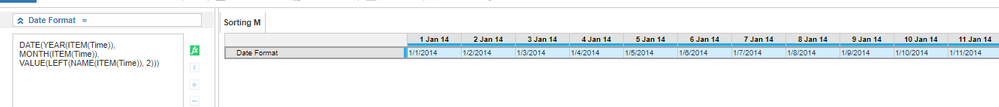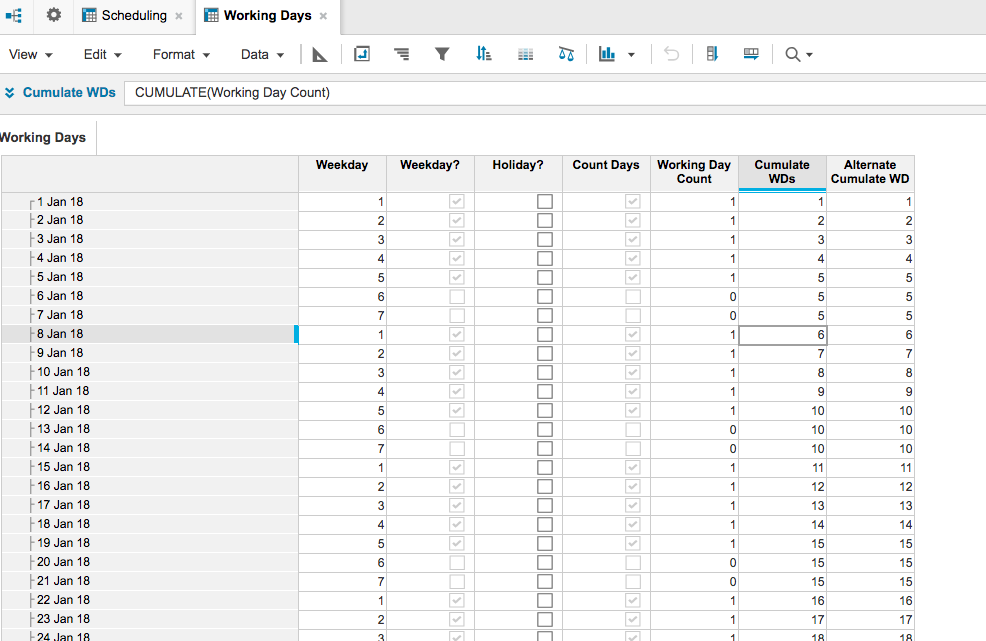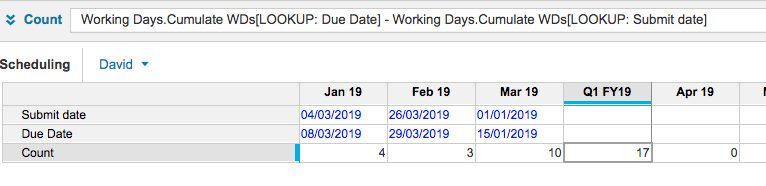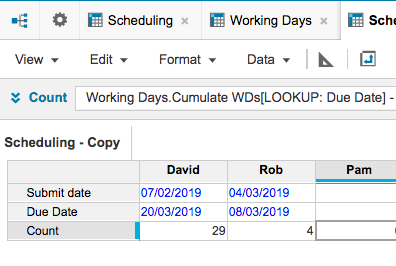# Time Dimension - dateHello,

Am trying to make a calendar in Anaplan to plot weekdays and holidays.

Time dimension for the model is "DAY" and I wanted not to type the dates in each days, but to put a formula instead. What would be the formula to return the "date" of the time dimension to the line item. I tried to use "ITEM(TIME)" but it does not return the date but has an error instead.

Sample: If the calendar date in the dimension is Jan 1, 2019, the line item would return 1/1/2019

I attached a screenshot of the calendar module I am working on.

Thank you!

Tagged:

•HI,

If your line item is formatted as text you can use the formula.

NAME(ITEM(TIME))

EDIT:

This formula seems to work, there's some interesting behaviour on the day function which needed some work around.

DATE(YEAR(ITEM(Time)), MONTH(ITEM(Time)), VALUE(LEFT(NAME(ITEM(Time)), 1)))

•HI Sara,

I understood, its very painful to do manual entry.

But it is really simple to bring the date in the line item. All you need to do is , make use of DATE function. If you try to write ITEM(TIME) it will work only if the format is time period. But here we are using DATE as a format, so please have a look at below screen shot for refrence.

I agree with @joshuastockwell but at last it should be 2 instead 1 because we do have 2 digits days uptil 31.I hope this really helps and solve your problem.

Thanks,

Kavin.

•Thank you so much! This helped 🙂
•Thank you for the response 🙂 appreciated 🙂
•Yes, it makes sense to use 2 instead of 1. Thank you so much! 🙂
•Not sure if my reply got lost in the mix, but just to re-emphasise, you don't need any complex formula here

START() will return the first day of the time period

END() will return the last day

It's as simple as that

David

••From the original attachment, I believe the data was at the day level, thus start() would work great.

Thanks,

Rob

•Another question re TIME DIMENSION. I have this encounter on calculation of working days, when I created a module with PERIOD as line item, the "count" line item formula produces result, whereas, if i use PERIOD as a TIME DIMENSION, the "count" formula in my line item does not produce any correct result. Do you know why if I use a time dimension, the formula should have a different approach?
•Could you post some screen shots of the modules and line items.  It would help to understand the detail more fully

Thanks

David

•Yes, the long formula worked. I needed a "per day" date in my line item that's why I didn't used Start () or end () and used the long formula instead. Thanks everyone for the help 🙂

@kavinkumar wrote:
Hi @david,

The above formula that you mentioned will work only to return 1st and last day of the month. But here the requirement is to get each date across the Time period.

@sarahcorrect me if I am wrong!!
Thanks,
Kavin.

•@rob_marshall wrote:

From the original attachment, I believe the data was at the day level, thus start() would work great.

Thanks,

Rob

yes! start() formula also worked 🙂 thanks

•Hi @DavidSmith attached is the screenshot, thank you 🙂

•You have a couple of things going on in here.  First, the formula.  Start() is resolving the date that you are currently looking at, in this case Jan 19.  So, in this case, what the formula is saying, if 1/1/19 is greater than 3/4/2019 and 1/31/2019 is less than 3/8/2019 then get the working days count from the Calendar module.

So, a couple of issues:  One, Jan 1st is not greater than 3/4/2019 while Jan 31st is less than 3/8/2019.  In using the AND, this will not resolve to a true.  Second issue: your Count module is at the day level while the Deadline module is at the Month level, the number that will be returned will be at the Month level (so not the highlighted values you have in the doc).

Hope this helps,

Rob

•Can you explain exactly what the use case and what you are trying to achieve?

you seem to have a time vs time construct, so it would be useful to know what you are expecting to see; that will hepl define the solution

David

•Hi @DavidSmith here you go, hope this helps for you to understand my question better 🙂

Thank you!

•Yes, thanks, that was the missing piece

As ever I think the solution is quite simple

Your holiday calendar is perfect; you were almost there, and with an extra line item we can solve itI've created two alternatives for the cumulative calculation  If you timescale is large, the alternative one is more efficient

But the line item formulae are:

Cumulate WDs = CUMULATE(Working Day Count)

Alternate Cumulate WD = Working Day Count + PREVIOUS(Alternate Cumulate WD)

Then in your target module you lookup the running total for the Due Date and subtract the running total from the Submit Date

Excuse my date format, but I'm based in the UK!!One question I did have and I've assumed it above is that you do need to different dates per month.  If that is not the case you can removed the time dimension from the module entirely, as belowI hope that solves it for you

David